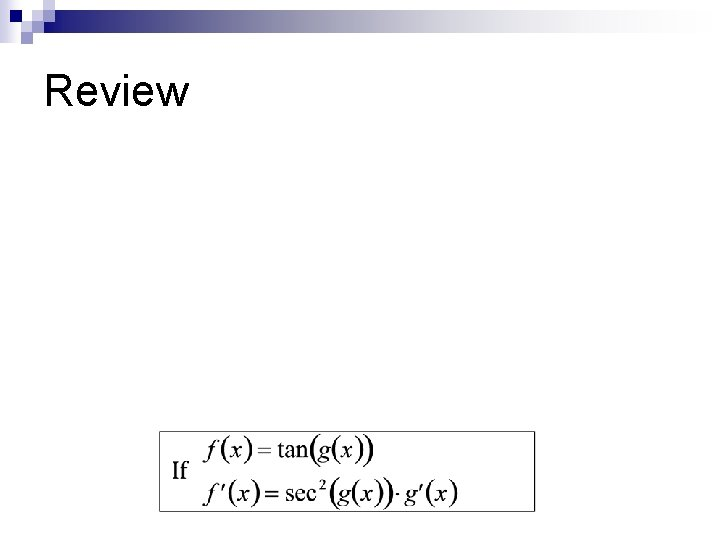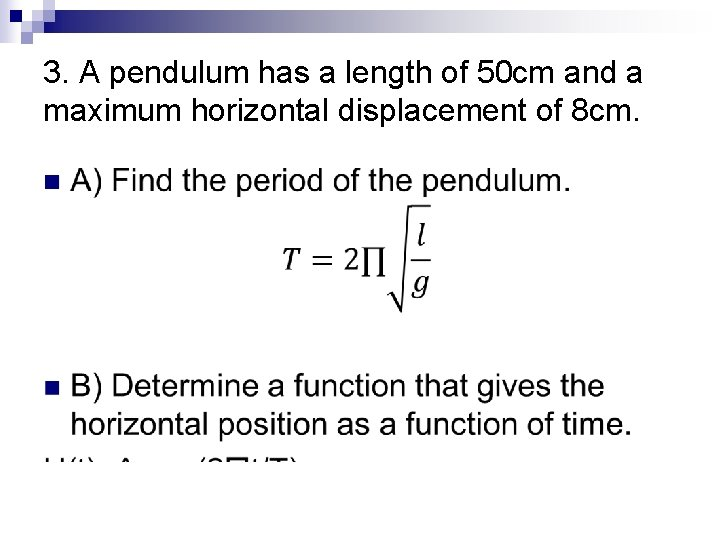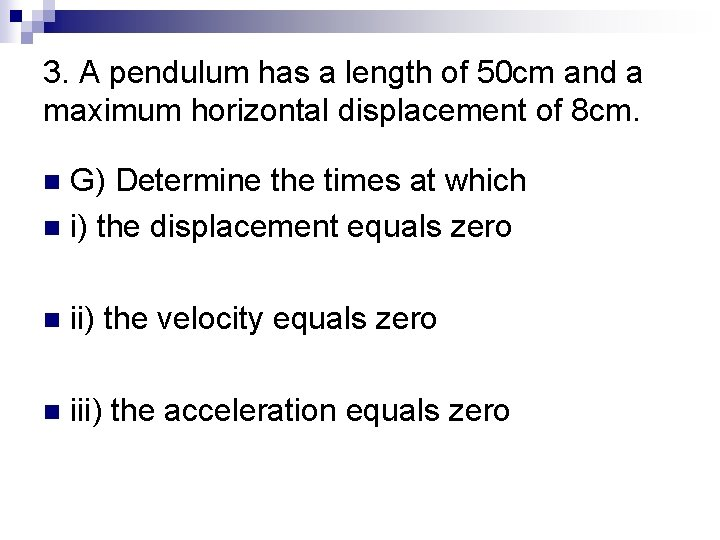# Unit 4 Applications of Trigonometric Functions Learning Goal

• Slides: 13Unit 4 Applications of Trigonometric FunctionsLearning Goal/ Big Idea n Learning Goal: Students will apply the derivatives of Sinusoidal functions into real life problems n Big Idea: The same algebraic expression or equation can be related to different real-world situations, and different algebraic expressions or equations can describe the same real-world situations.Minds On n Why derivatives of a sinusoidal function?ReviewApplications of Sinusoidal Functions 1. A power supply delivers a voltage signal that consists of an alternating current (AC) component and a direct current (DC) component. Where t is the time, in seconds, the voltage, in volts, at time t is given by the function V(t)=5 sint+12 a) Find the maximum and minimum voltages. At which times do these values occur? b) Determine the period, T, in seconds, frequency, f, in hertz, and amplitude, A, in volts, for this signal2. A piston in an engine oscillates up and down from a rest position. The motion of the piston can be approximated by the function h(t)=0. 05 cos(13 t), where t is time, in seconds, and h is the displacement of the piston head from rest position, in meters, at time t. n A) Determine an equation for the velocity of the piston head as a function of time n B) Find the maximum and minimum velocities and the times at which they Occur.3. A pendulum has a length of 50 cm and a maximum horizontal displacement of 8 cm. n3. A pendulum has a length of 50 cm and a maximum horizontal displacement of 8 cm. n C) Determine a function that gives the velocity of the bob as a function of time. n D) Determine a function that gives the acceleration of the bob as a function of time.3. A pendulum has a length of 50 cm and a maximum horizontal displacement of 8 cm. n E) Find the maximum velocity of the bob and when it occurs.3. A pendulum has a length of 50 cm and a maximum horizontal displacement of 8 cm. n F) Find the maximum acceleration of the bob and when it first occurs.3. A pendulum has a length of 50 cm and a maximum horizontal displacement of 8 cm. G) Determine the times at which n i) the displacement equals zero n n ii) the velocity equals zero n iii) the acceleration equals zeroExit Ticket n Find the derivative of f(x)= cotx